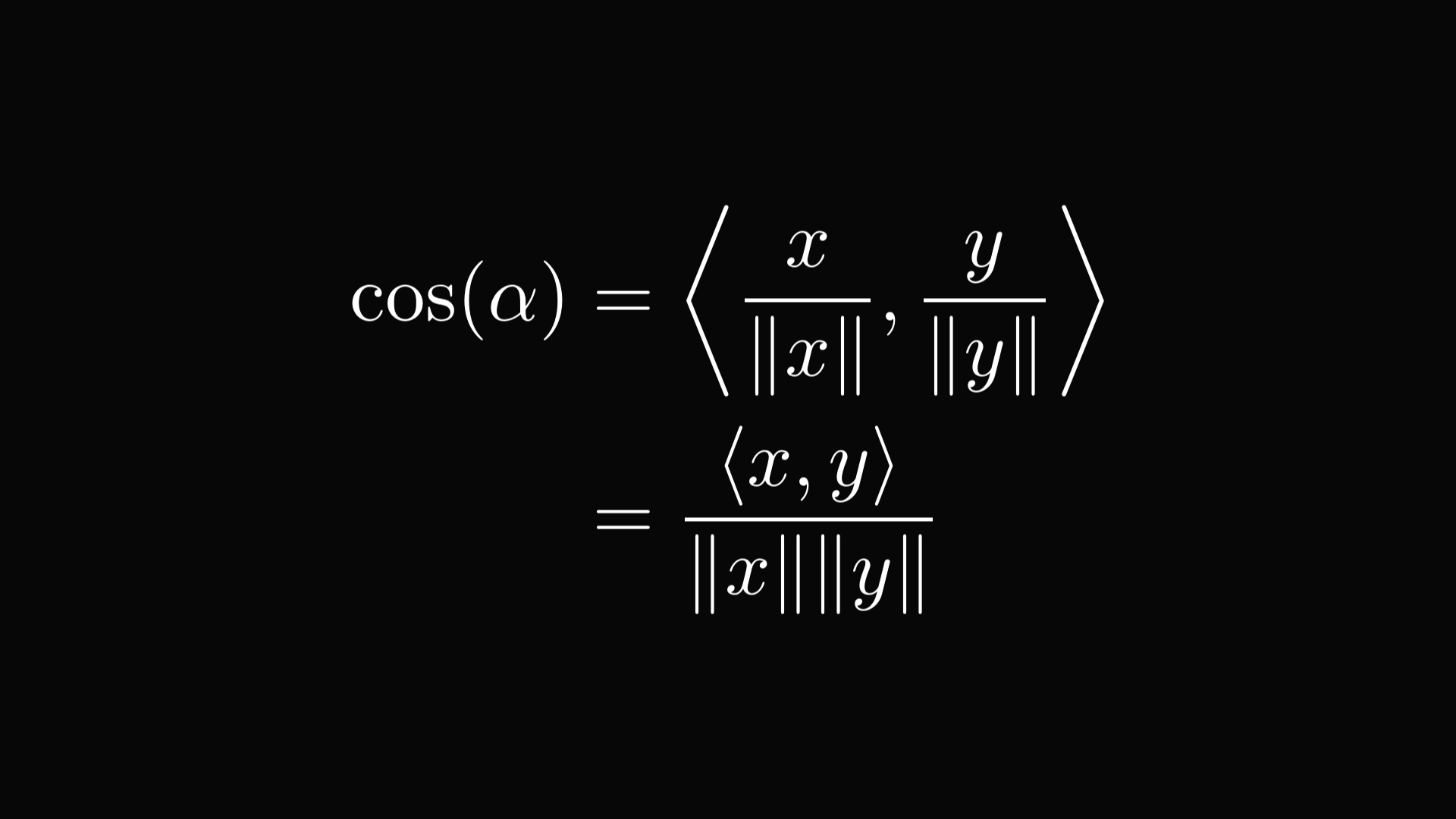# How the dot product measures similarity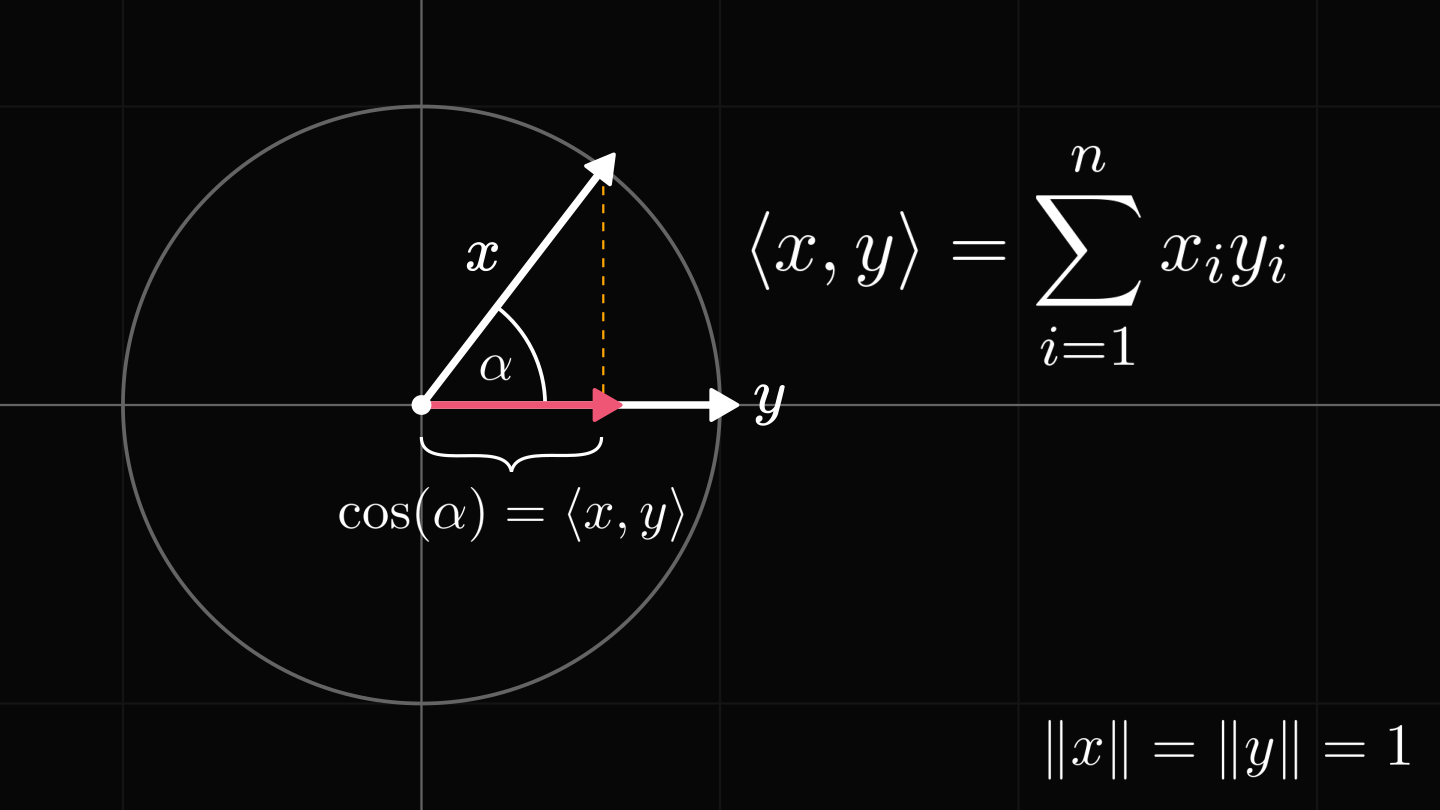In machine learning, we use the dot product every day.

Its definition is far from revealing. For instance, what does the sum of coordinate products have to do with similarity?

There is a beautiful geometric explanation behind.

The dot product is one of the most fundamental concepts in machine learning, making appearances almost everywhere. By definition, the dot product (or inner product) is defined between two vectors as the sum of coordinate products.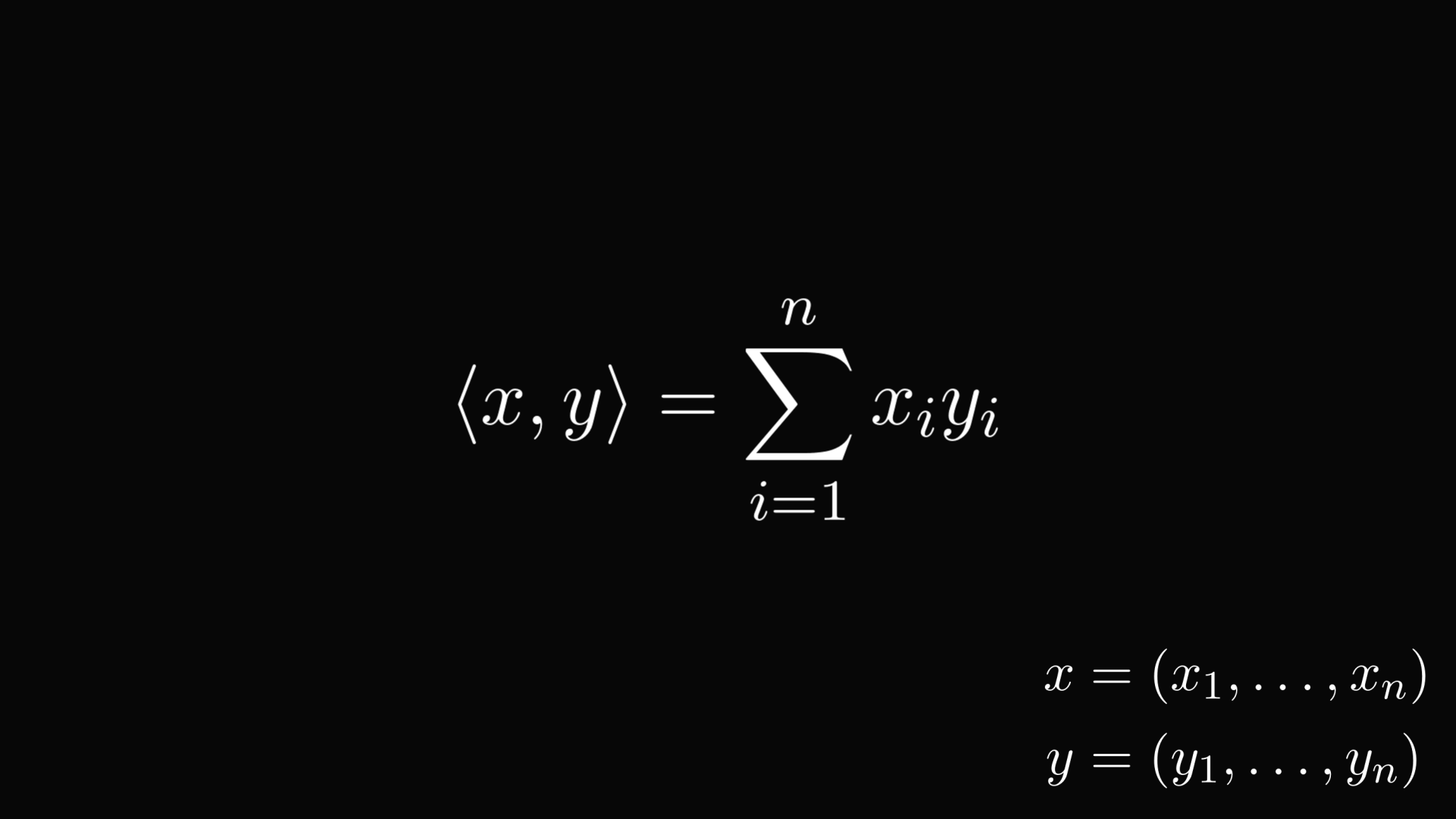## The fundamental properties of the dot product

To peek behind the curtain, there are three key properties that we have to understand.

First, the dot product is linear in both variables. This property is called bilinearity.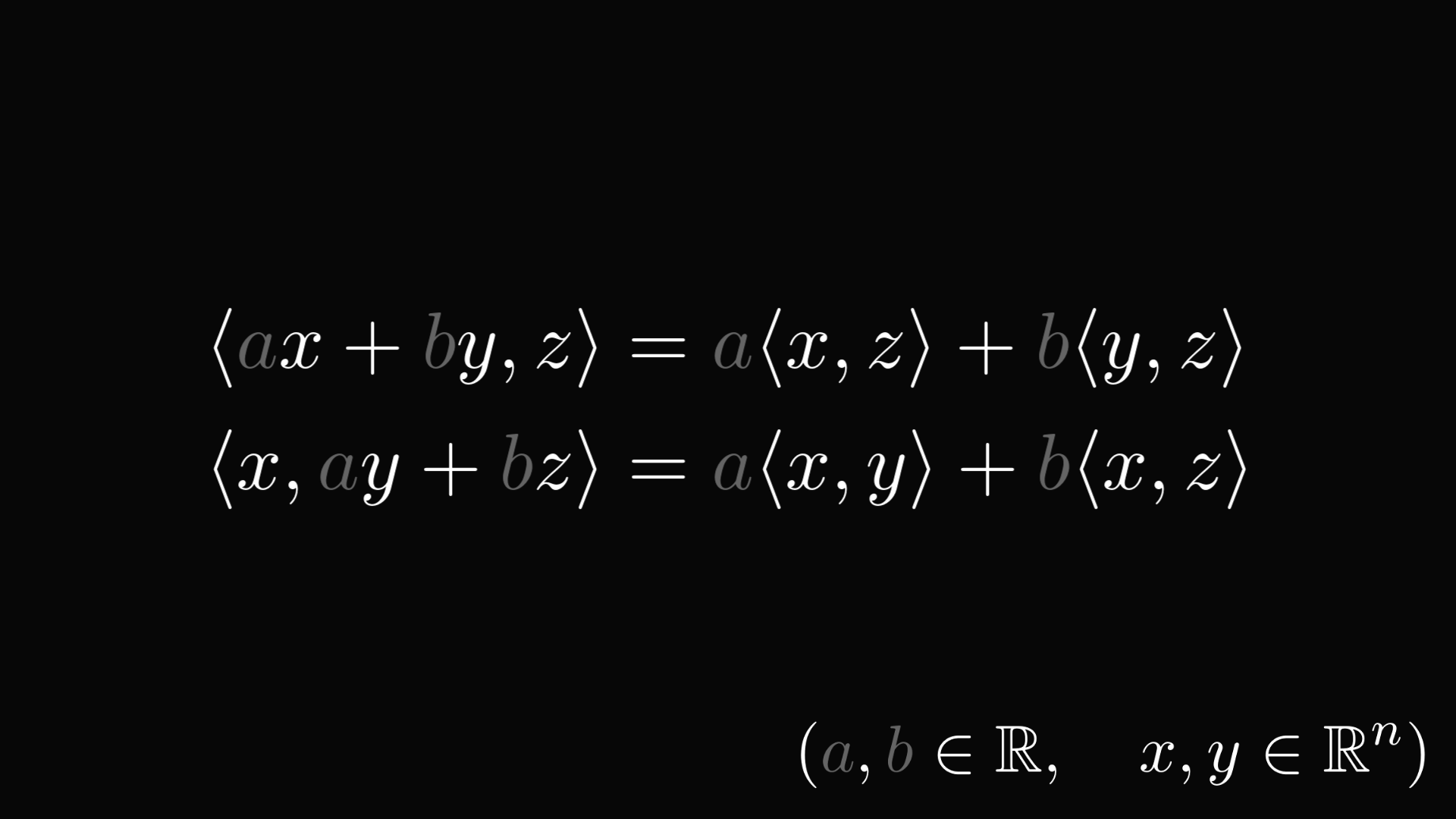Second, the dot product is zero if the vectors are orthogonal. (In fact, the dot product generalizes the concept of orthogonality beyond Euclidean spaces. But that's for another day :) )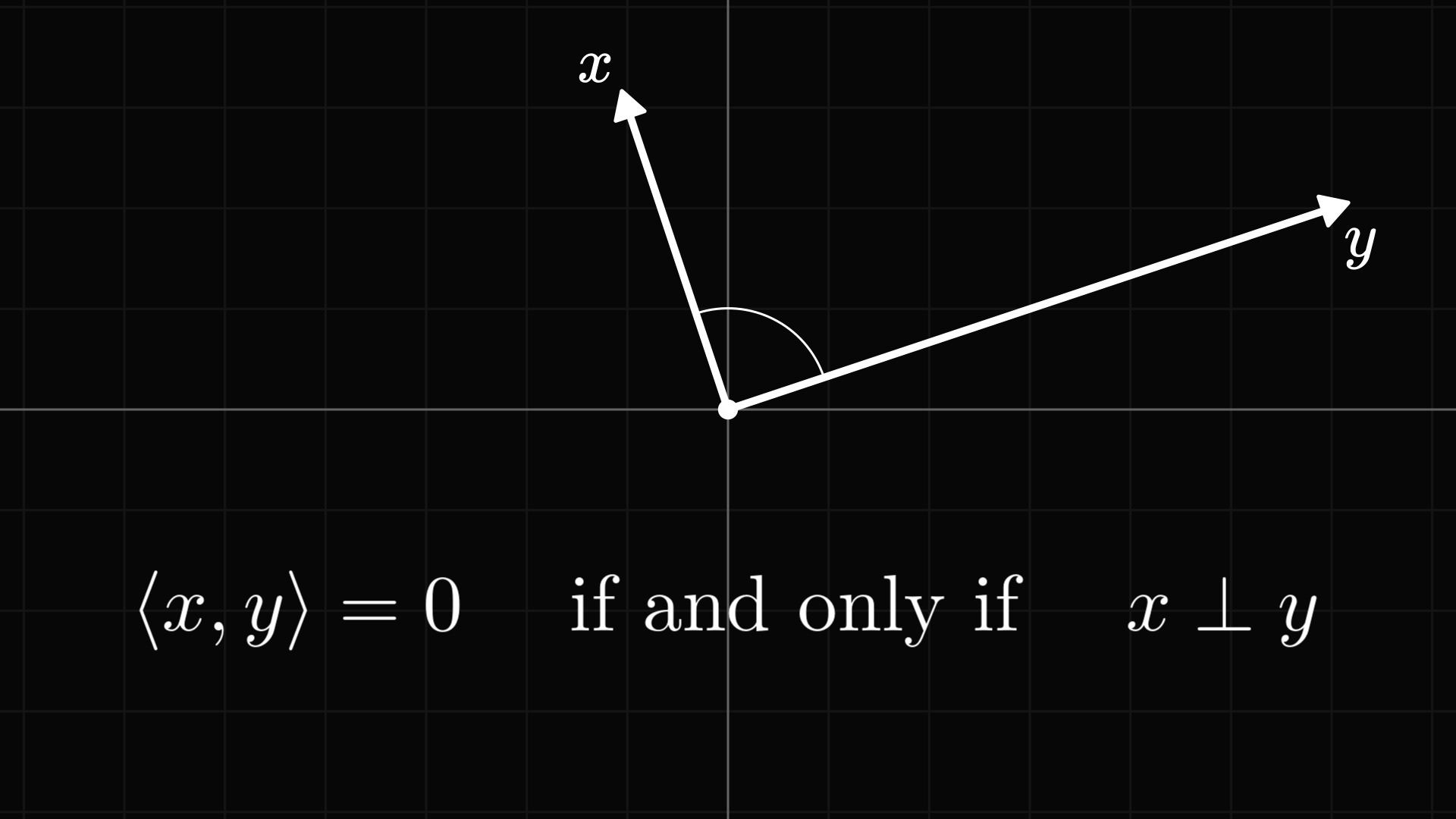Third, the dot product of a vector with itself equals the square of its magnitude.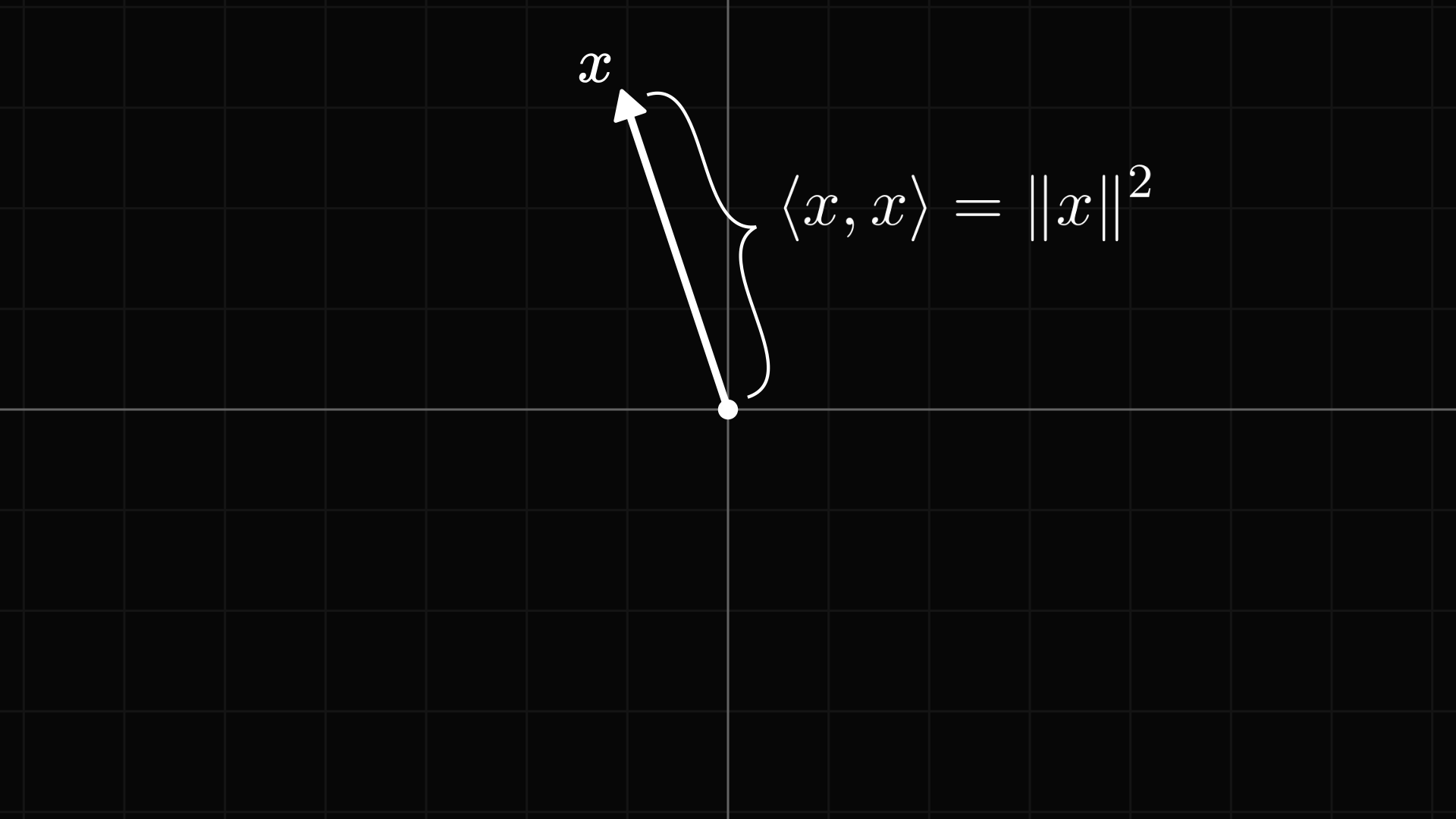## The geometric interpretation of the dot product

Now comes the interesting part. Given a vector $y$, we can decompose $x$ into the two components $x_o$ and $x_p$. One is parallel to $y$, while the other is orthogonal to it.

In physics, we apply the same decomposition to various forces all the time.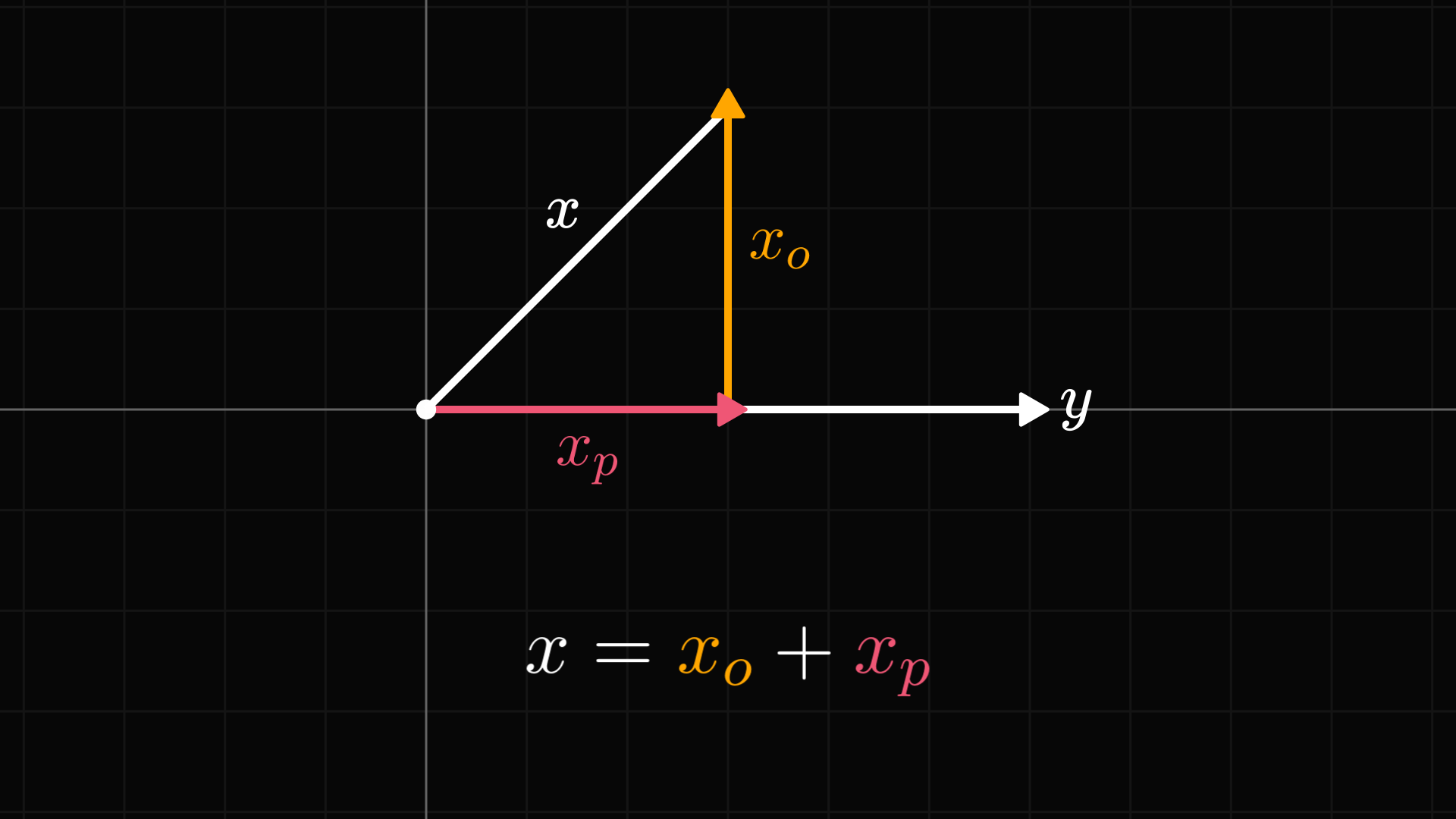The vectors $x_o$ and $x_p$ are characterized by two properties:

1. $x_p$ is a scalar multiple of $y$,
2. and $x_0$ is orthogonal to $x_p$ (and thus to $y$).

We are going to use these properties to find an explicit formula for $x_p$. Spoiler alert: it is related to the dot product.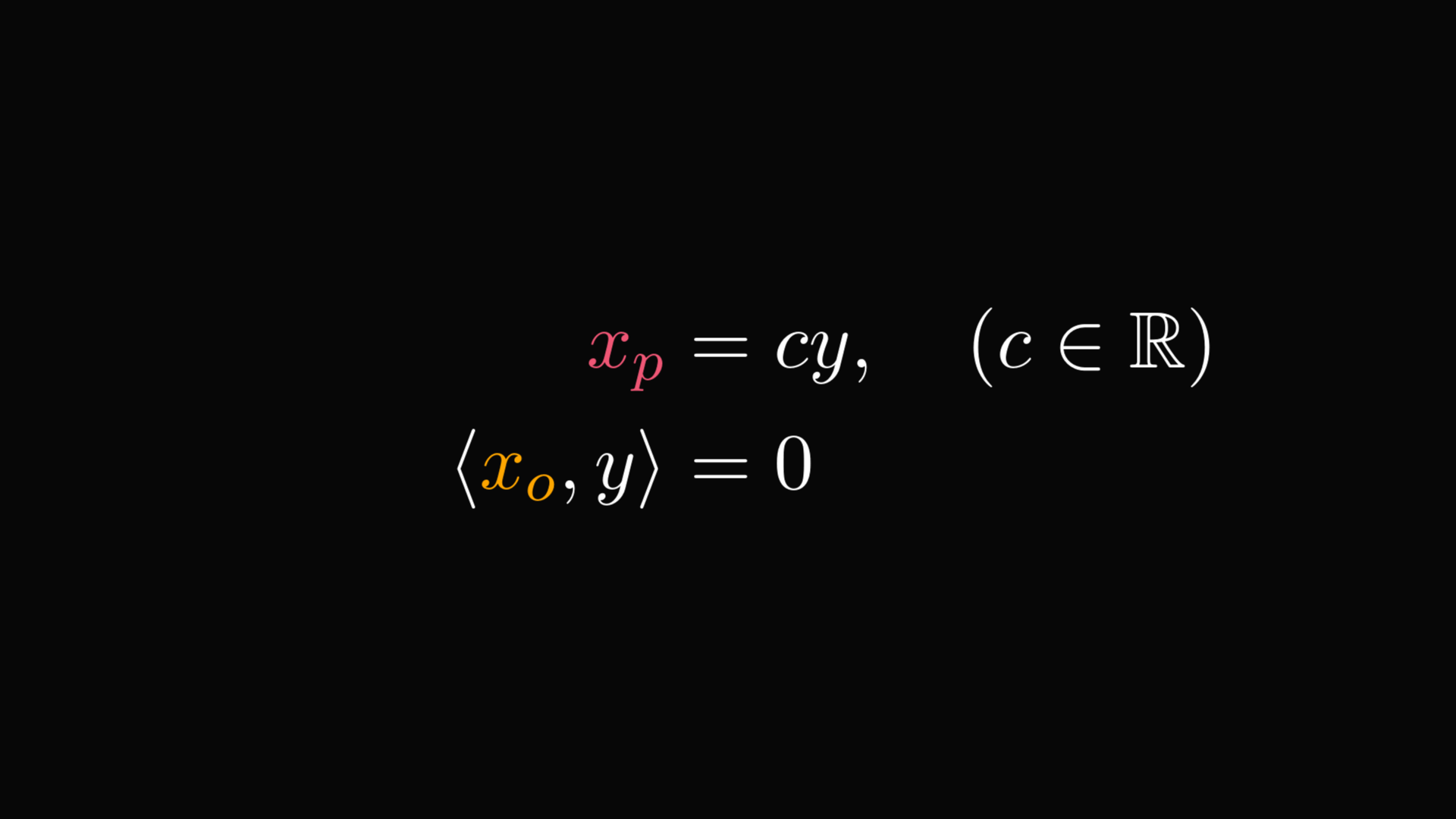Due to $x_0$ being orthogonal to $y$, we can use the bilinearity of the dot product to express the $c$ in $x_p = c y$.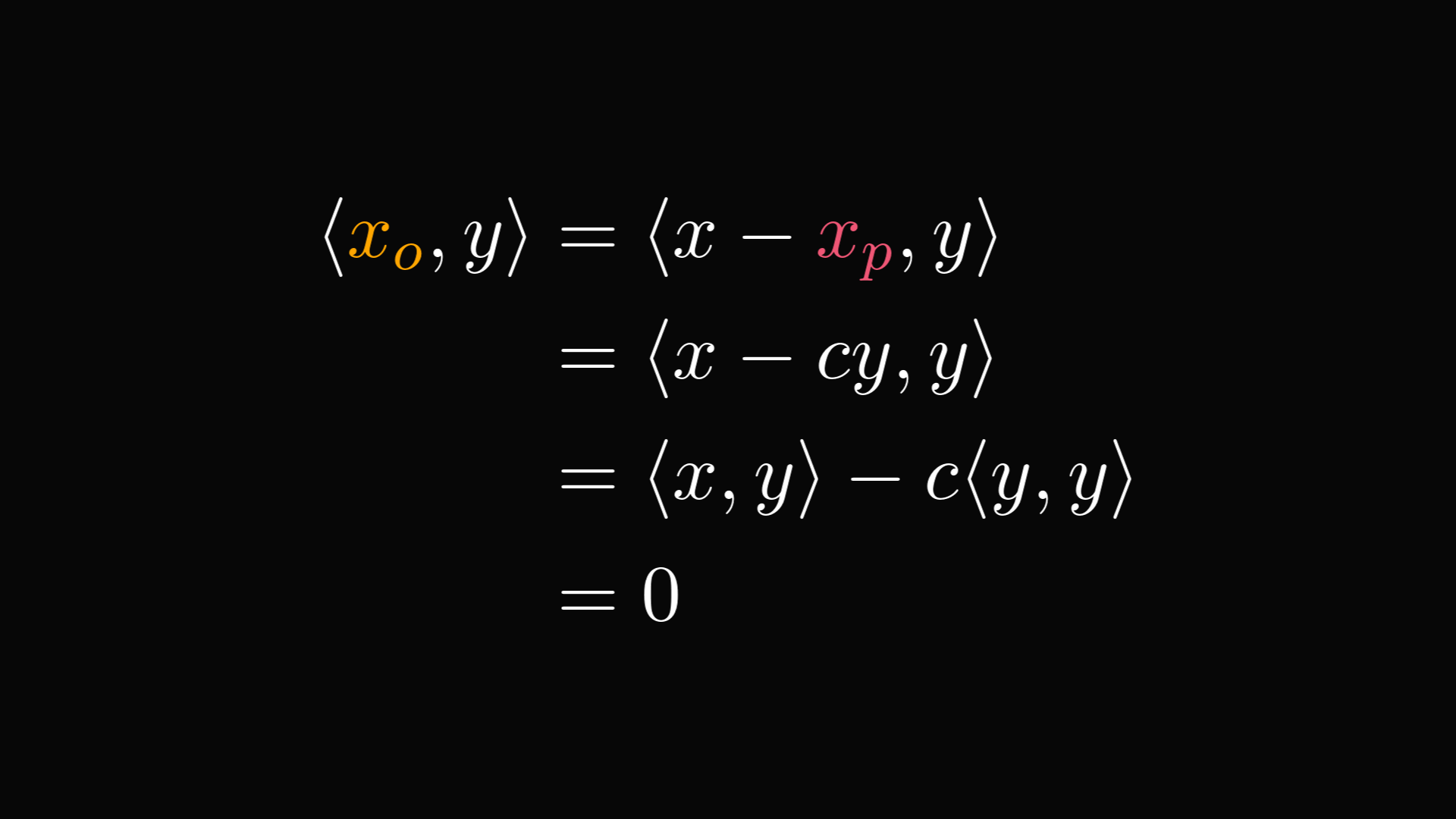By solving for $c$, we get that it is the ratio of the dot product and the magnitude of $y$.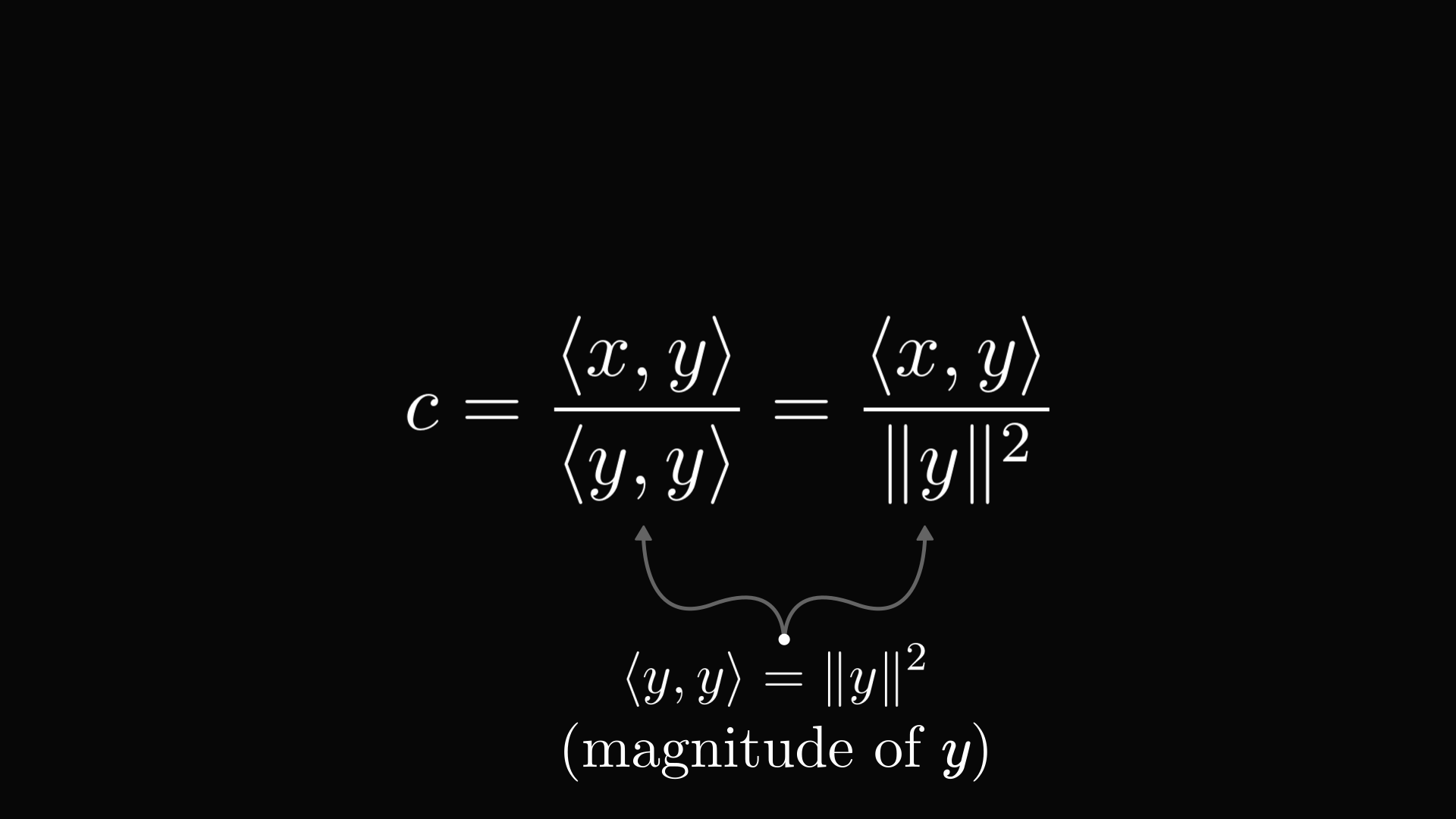If both $x$ and $y$ are unit vectors, the dot product simply expresses the magnitude of the orthogonal projection!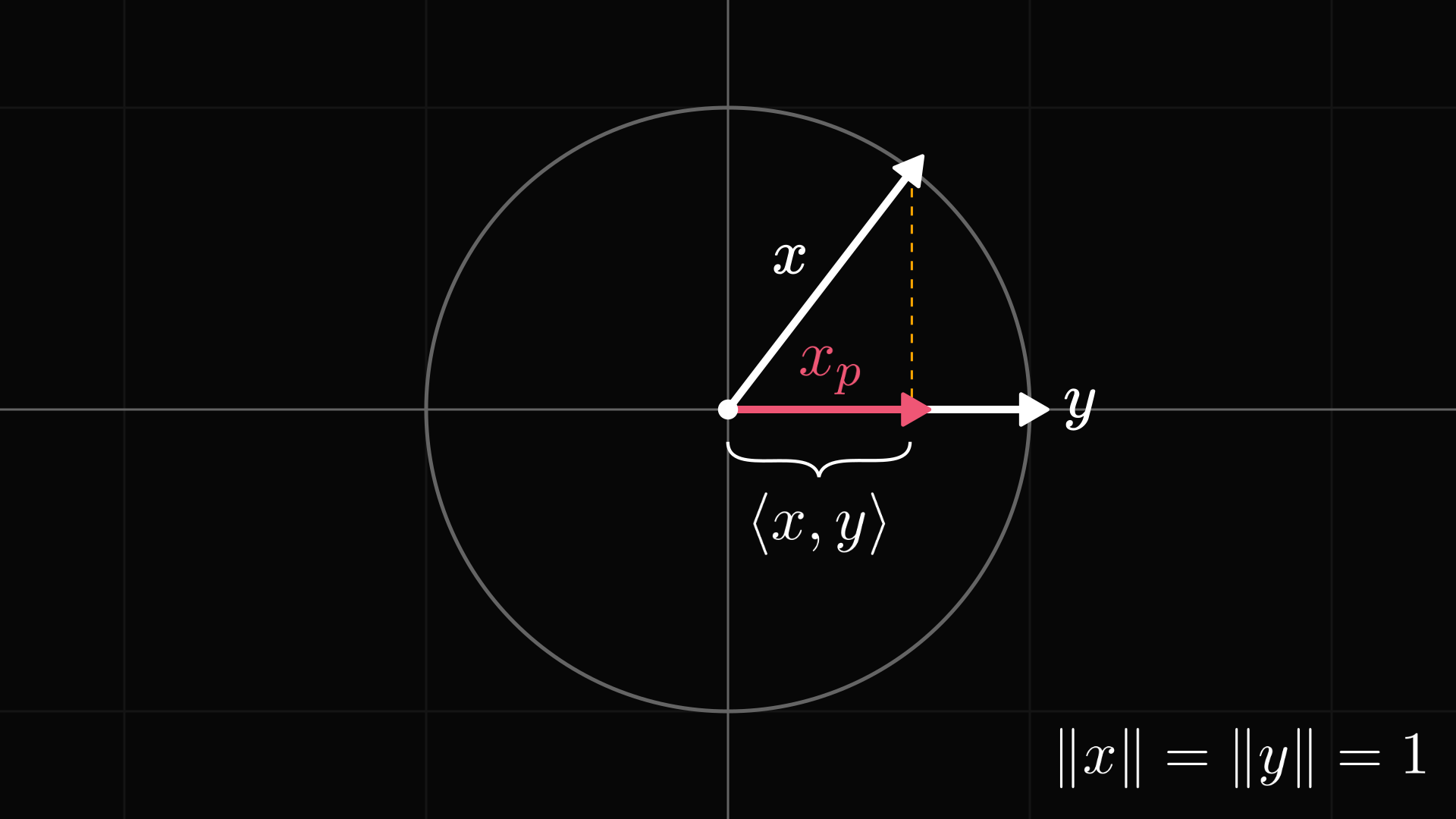## Dot product as similarity

Do you recall how the famous trigonometric functions sine and cosine are defined?

Let's say that the hypotenuse of our right triangle is a unit vector and one of the legs is on the $x$-axis. Then the trigonometric functions equal the magnitudes of the projections to the axes.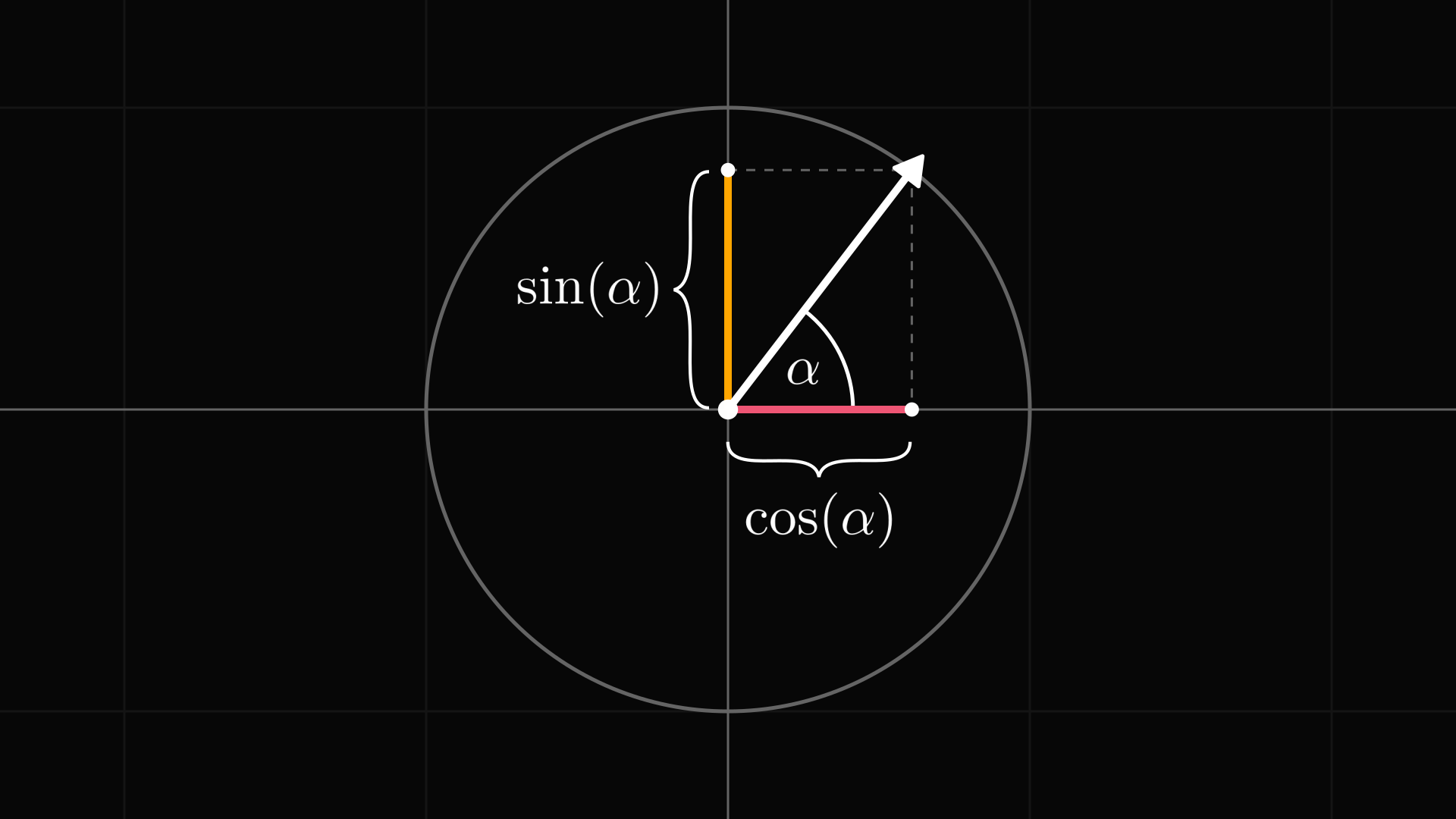Using trigonometric functions, we see that the dot product of two unit vectors is the cosine of their enclosed angle $\alpha$! This is how the dot product relates to cosine.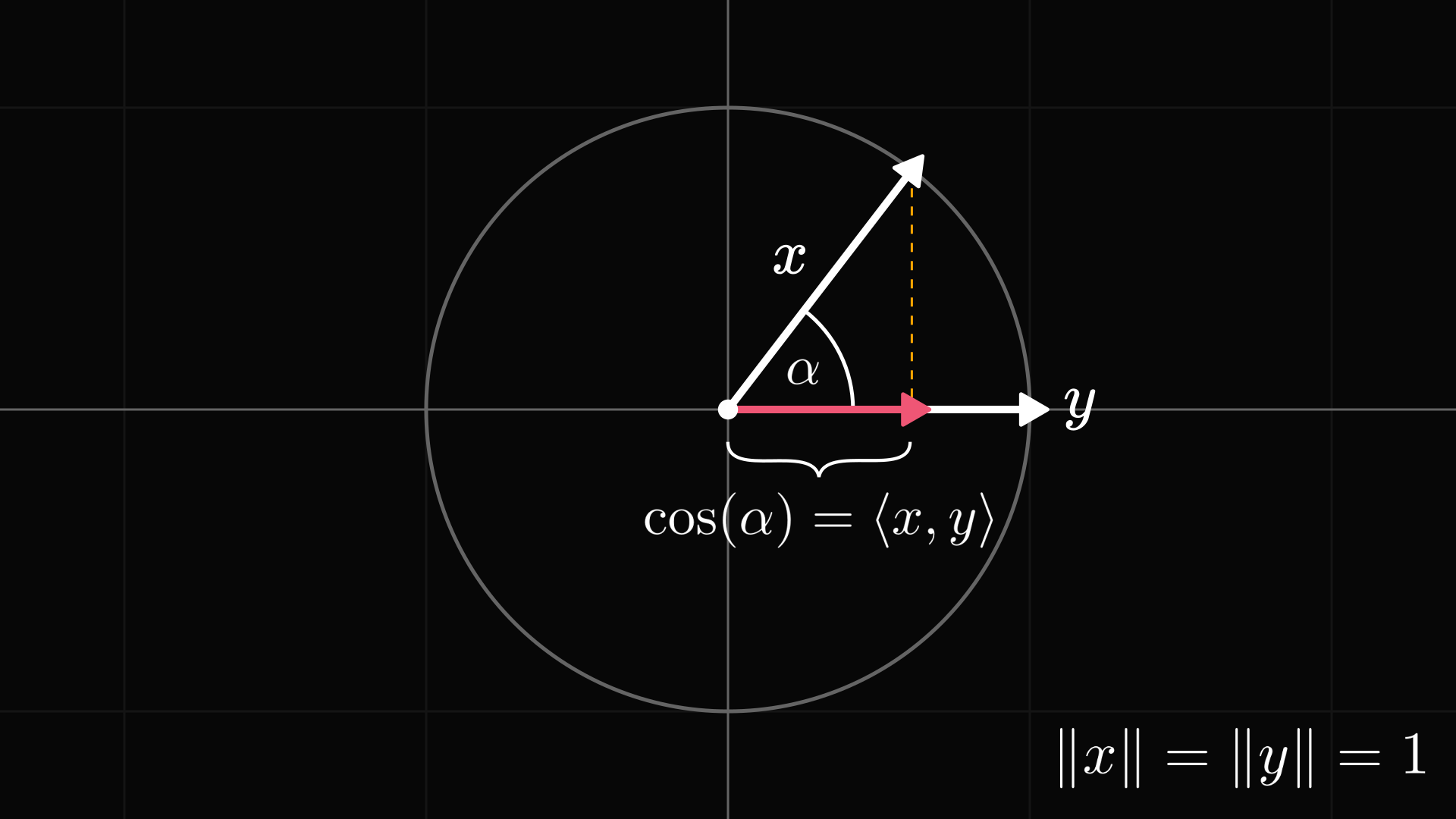If $x$ and $y$ are not unit vectors, we can scale them and use our previous discovery to get the cosine of $\alpha$.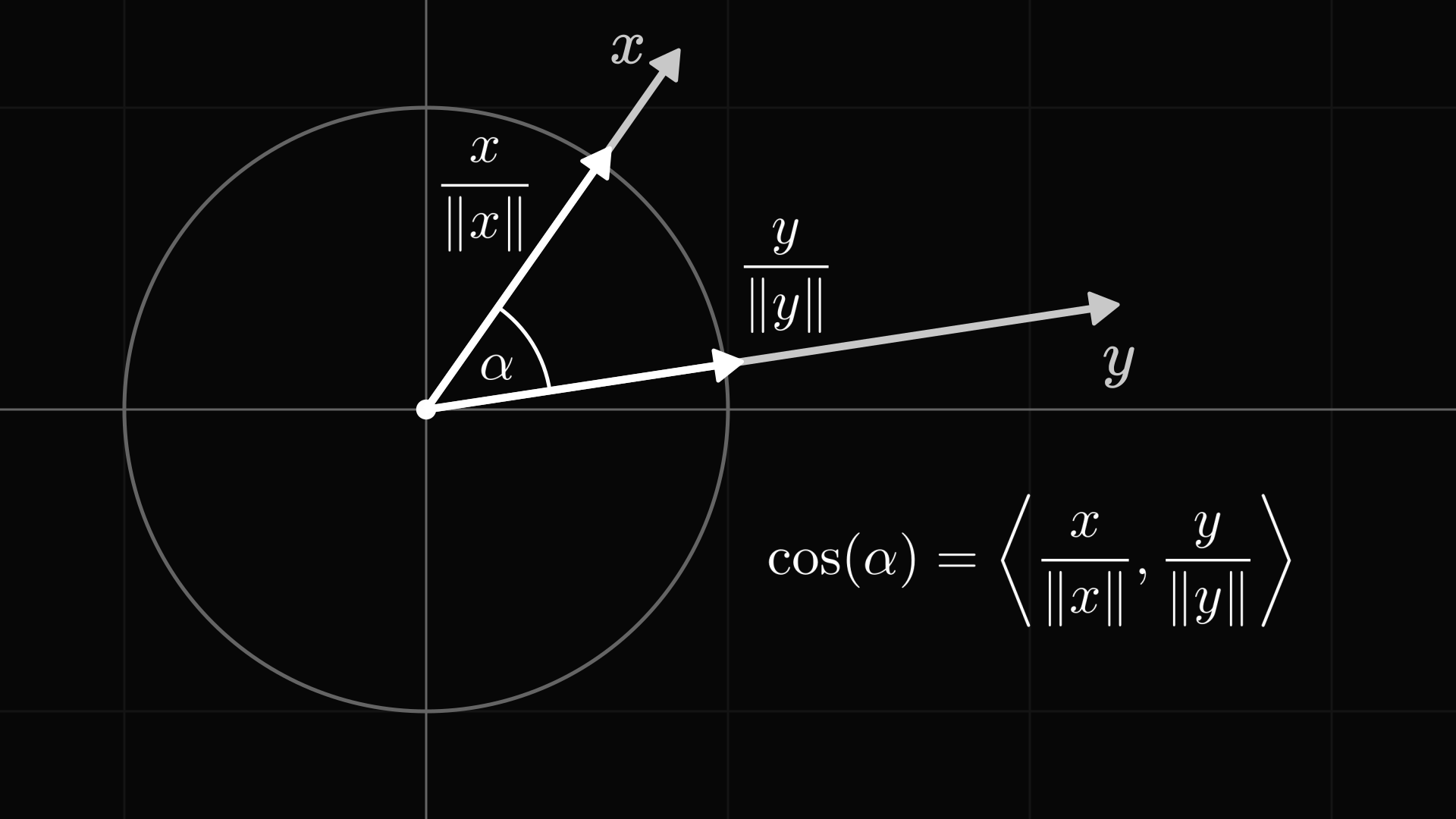The closer to its value to $1$, the more similar $x$ and $y$ are. (In a sense.) In machine learning, we call this quantity the cosine similarity.

Now you understand why.